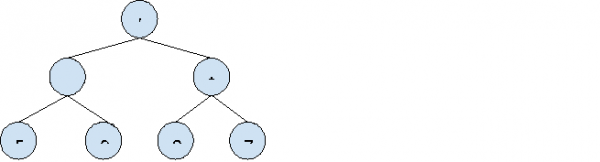# Evaluation of Expression Tree in C++

In this problem, we are given an expression tree that consist of binary operations like +, - , /, *. We need to do the evaluation of the expression tree and then return the result.

Expression Tree is a special type of binary tree in which each node either consist of an operator or operand which are distributed as−

• Leaf nodes of the tree are values on which the operation is to be performed.
• Non-leaf nodes consist of the binary operator denoting the operation to be performed.

## Let’s take an example to understand the problem,

Input:Output: 1

Explanation:

Decoding the expression tree,

Exp             = ( (5+9) / (2*7) )
= ( 14 / 14 )

= 1

## Solution Approach:

A simple solution to the problem is by performing one operation each from root, for operends we will solve the subtree. As all operations are binary the nodes of a tree either have two childrens or none.

We will use recursion to solve each node's binary operation.

## Example

Live Demo

#include <bits/stdc++.h>
using namespace std;

class node {

public:
string value;
node *left = NULL, *right = NULL;
node(string x)
{
value = x;
}
};

int solveExpressionTree(node* root) {

if (!root)
return 0;

if (!root->left &amp;&amp; !root->right)
return stoi(root->value);

int leftSubTreeSol = solveExpressionTree(root->left);
int rightSubTreeSol = solveExpressionTree(root->right);

if (root->value == "+")
return leftSubTreeSol + rightSubTreeSol;

if (root->value == "-")
return leftSubTreeSol - rightSubTreeSol;

if (root->value == "*")
return leftSubTreeSol * rightSubTreeSol;

if (root -> value == "/")
return leftSubTreeSol / rightSubTreeSol;

return -1;
}

int main()
{
node *root = new node("/");
root->left = new node("+");
root->left->left = new node("9");
root->left->right = new node("5");
root->right = new node("*");
root->right->left = new node("2");
root->right->right = new node("7");
cout<<"The evaluation of expression tree is "<<solveExpressionTree(root);
return 0;
}

## Output −

The evaluation of expression tree is 1